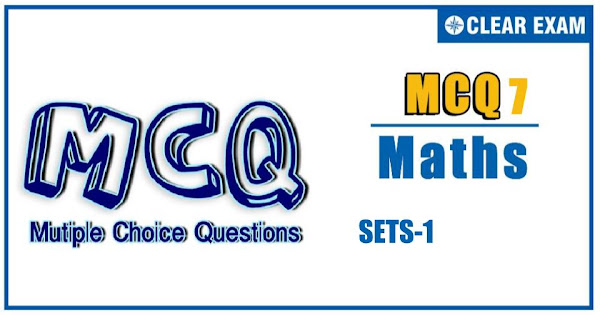## SETS-1 Quiz-7

In mathematics a set is a collection of distinct elements. The elements that make up a set can be any kind of things: people, letters of the alphabet, numbers, points in space, lines, other geometrical shapes, variables, or even other sets. Two sets are equal if and only if they have precisely the same elements. Sets are ubiquitous in modern mathematics. Indeed, set theory, more specifically Zermelo–Fraenkel set theory, has been the standard way to provide rigorous foundations for all branches of mathematics since the first half of the 20th century..

Q1. If A={a,b,c,l,m,n}, then the maximum number of elements in any relation on A is
•  12
•  16
•  32
•  36
Solution
(d) Any relation on A is a subset of A×A which contains 36 elements. Hence, maximum number of elements in a relation on A can be 36

Q2.Let A be the set of all animals. A relation R is defined as "aRb iff a and b are in different zoological parks”. Then R is
•  Only reflexive
•  Only symmetric >
•   Only transitive
•  Equivalence
Solution
(b) Clearly, (a,a)∈R for any a∈A Also, (a,b)∈R ⇒a and b are in different zoological parks ⇒b and a are in different zoological parks ⇒(b,a)∈R Now, (a,b)∈R and (b,a)∈R but (a,a)∉R So, R is not transitive

Q3.  In a class of 35 students, 17 have taken Mathematics, 10 have taken Mathematics but not Economics. If each student has taken either Mathematics or Economics or both, then the number of students who have taken Economics but not Mathematics is
•   7
•  25
•  18
•  32
Solution
(c) Let M and E denote the sets of students who have taken Mathematics and Economics respectively. Then, we have n(M∪E)=35,n(M)=17 and n(M∩E^' )=10 Now, n(M∩E^' )=n(M)-n(M∩E) ⇒10=17-n(M∩E)⇒n(M∩E)=7 Now, n(M∪E)=n(M)+n(E)-n(M∩E) ⇒35=17+n(E)-7⇒n(E)=25 ∴n(E∩M^' )=n(E)-n(E∩M)=25-7=18

Q4. A and B are any two non-empty sets and A is proper subset of B. If n(A)=5, then find the minimum possible value of n(A∆B)
•  Is 1
•  Is 5
•  Cannot be determined
•  None of these
Solution
(a) It is given that A is a proper subset of B ∴A-B=ϕ⇒n(A-B)=0 We have, n(A)=5. So, minimum number of elements in B is 6 Hence, the minimum possible value of n(A Δ B) is n(B)-n(A)=6-5=1

Q5. If n(A_i )=i+1 and A_1⊂A_2⊂A_3⊂⋯⊂A_99, then n(⋃_(i=1)^99▒A_i )=
•  99
•  98
•  100
•  101
Solution
(c) It is given that A_1⊂A_2⊂A_3…⊂A_99 ⋃_(i=1)^999▒〖A_i=A_99 〗 ⇒n(⋃_(i=1)^99▒A_i )=n(A_99 )=99+1=100

Q6. If S is a set with 10 elements and A={(x,y):x,y∈S,x≠y}, then the number of elements in A is
•  100
•  90
• 50
•  45
Solution
(b) Number of element is S=10 And A={(x,y);x,y∈S,x≠y} ∴ Number of element in A=10×9=90

Q7. Let X={1,2,3,4,5} and Y={1,3,5,7,9}. Which of the following is/are not relations from X to Y?
•  R_1={(x,y)|y=2+x,x∈X,y∈Y}
•  R_2={(1,1),(2,1),(3,3),(4,3),(5,5)}
•  R_3={(1,1),(1,3),(3,5),(3,7),(5,7)}
•  R_4={(1,3),(2,5),(2,4),(7,9)}
Solution
(d) R_4 is not a relation from A to B, because (7,9)∈R_4 but (7,9)∉A×B

Q8. If A is a finite set having n elements, then P(A) has
•  2n elements
•  T2^n elements
•  n elements
•  None of these
Solution
B

Q9. In the above question, the number of families which buy none of A,B and C is
•  4000
•  3300
•  4200
•  5000
Solution
(a) We have, Required number of families =n(A'∩B'∩C') =n(A∪B∪C)' =N-n(A∪B∪C) =10000-{n(A)+n(B)+n(C)-n(A∩B)} -n(B∩C)-n(A∩C)+n(A∩B∩C)} =10000-4000-2000-1000+500+300+400-200 =4000

Q10. If A⊆B, then B∪A is equal to
•  B∩A
•  A
•  B
• None of these
Solution
(c) ∵ A⊆B ∴ B∪A=B#### Written by: AUTHORNAME

AUTHORDESCRIPTION## Want to know more

Please fill in the details below:

## Latest NEET Articles\$type=three\$c=3\$author=hide\$comment=hide\$rm=hide\$date=hide\$snippet=hide

Name

ltr
item
BEST NEET COACHING CENTER | BEST IIT JEE COACHING INSTITUTE | BEST NEET & IIT JEE COACHING: Sets-1-Quiz-7
Sets-1-Quiz-7
https://1.bp.blogspot.com/-SQwVYs8eUTA/YNyWY2oJRCI/AAAAAAAAI9M/DwrHHw6YY3UmG1LMA4C1Ca8mlz1B3e6BwCLcBGAsYHQ/s600/Quiz%2BImage%2B20%2B%25282%2529.jpg
https://1.bp.blogspot.com/-SQwVYs8eUTA/YNyWY2oJRCI/AAAAAAAAI9M/DwrHHw6YY3UmG1LMA4C1Ca8mlz1B3e6BwCLcBGAsYHQ/s72-c/Quiz%2BImage%2B20%2B%25282%2529.jpg
BEST NEET COACHING CENTER | BEST IIT JEE COACHING INSTITUTE | BEST NEET & IIT JEE COACHING
https://www.cleariitmedical.com/2021/07/sets-1-quiz-7.html
https://www.cleariitmedical.com/
https://www.cleariitmedical.com/
https://www.cleariitmedical.com/2021/07/sets-1-quiz-7.html
true
7783647550433378923
UTF-8

STAY CONNECTED